### Given the following traverse data:Figure G-1Example Problem

### Explain the computation process to determine:

• Coordinates of the traverse points
• Area of the traverse

### Compute Coordinates

 Start at point D; perform a forward computation to point C.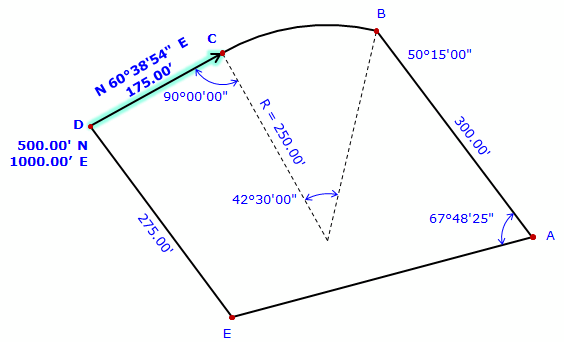At point C, compute direction to radius point; Az = (60°38’54”+180°)-90°00’00” = 150°38’54” Perform a forward computation to radius point.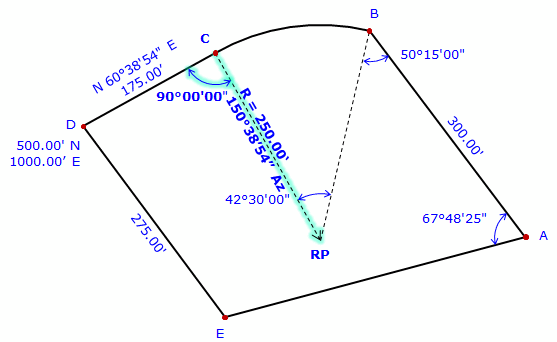At radius point, compute direction to point B. Az = (150°38'54"+180°00'00") + 42°30'00" = 373°08'54" = 13°08'54" Perform a forward computation to point B.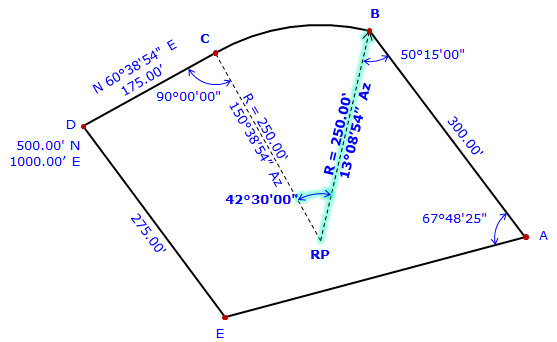At point B, compute direction to point A. Az = (13°08'54"+180°00'00) - 50°15'00" = 142°53'54" Perform a forward computation to point A.There isn't sufficient data to perform a forward computation from point A to point E. Point E is also connected to point D but there isn't enough data to perform a forward computation from point D. However, point E can be determined by a Distance-Direction intersection using its connections to points A and D. Compute direction from point A to E Az = (142°53'54"+180°00'00") - 67°48'25" = 255°05'09" Compute the Distance-Direction intersection.Compute Area

#### Method 1

 List coordinates through the radius point and compute the area.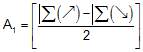Compute the Sector area.Add the two areas together to determine total area: Area = A1 + A2

#### Method 2

Compute along chord

 List coordinates going along the chord and compute the area.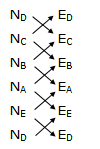Compute the Segment area.Add the two areas together to determine total area: Area = A1 + A2